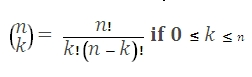# SQL Server binomial coefficient function

Updated: 9 August 2010
Use BICO to calculate the binomial coefficient. This is the equivalent of the COMBIN function in the XLeratorDB/math moduleSyntax
SELECT [wctStatistics].[wct].[BICO] (
<@N, float,>
,<@K, float,>)
Arguments
@N
is any positive number. @N is an expression of type float or of a type that can be implicitly converted to float
@K
is any positive number. @K is an expression of type float or of a type that can be implicitly converted to float.
Return Types
float
Remarks
·         If @N <0, BICO returns an error.
·         If @K ≤0, BICO returns an error.
·         If @K > @N, BICO returns an error.
·         Both @N and @K are truncated to zero decimal places.
Examples

SELECT
wct.BICO(14, 3)

This produces the following result

----------------------
364

(1 row(s) affected)

### SupportCopyright 2008-2021 Westclintech LLC         Privacy Policy        Terms of Service